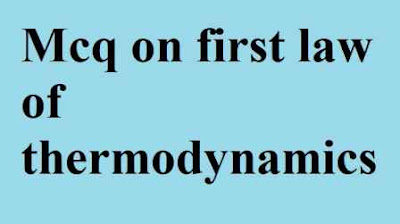### Mcq on First law of thermodynamics (objective questions and answers)

In mechanical engineering mcq website today we solving Thermodynamics  subject multiple choice questions Mcq on First law of thermodynamics (objective questions and answers)

Guys this first law of thermodynamics  in thermodynamics  mcq with answer is important for all competitive exam so without wasting time start Mcq on first law of thermodynamics

# Mcq on First law of thermodynamics (objective questions and answers)Mcq on First law of thermodynamics (objective questions and answers)

First law of thermodynamics mcq - 1
Which scientists discovered the first law of thermodynamics ?

A. Kelvin
B. Joule
C. plank
D. None of these

First law of thermodynamics mcq - 2
First law of thermodynamics define which thermodynamics properties  ?

A. Enthalpy
B. Entropy
C. internal energy
D. Power

First law of thermodynamics mcq - 3
First law of thermodynamics deal's about

A. Conservation of work
B. Conservation of heat
C. Conservation of mass
D. Conservation of energy

First law of thermodynamics mcq - 4
The internal energy is represented by

A. Q
B. W
C. U
D. H

First law of thermodynamics mcq - 5
What is si unit of internal energy ?

A. Joule
B. Pascal
C. Bar
D. Unit less quality

First law of thermodynamics mcq - 6
PMM 1 is Reversible of ........

A. Zeroth law of thermodynamics
B. First law of thermodynamics
C. Second law of thermodynamics
D. Third law of thermodynamics

First law of thermodynamics mcq - 7
Si Unit of radius of gyration is .......

A. meter
B. Second
C. meter ²
D. Newton

First law of thermodynamics mcq - 8
According to first law of thermodynamics the total energy of system is remains constant.

A. True
B. False

First law of thermodynamics mcq - 9
The moment of inertia of a square is a .....

A. a / 12
B. a² / 12
C. a³ / 12
D. a⁴ / 12

First law of thermodynamics mcq - 10
First law of thermodynamics is discovered by scientists Joule.

A. True
B. False

First law of thermodynamics mcq - 11
According to PMM 1 first law of thermodynamics is ..............

A. Possible
B. Impossible

First law of thermodynamics mcq - 12
First law of thermodynamics is laws of conservation of energy .

A. True
B. False

First law of thermodynamics mcq - 13
Which thermodynamics law is defined the Internal energy ?

A. Zeroth law of thermodynamics
B. First law of thermodynamics
C. Second law of thermodynamics
D. Third law of thermodynamics

First law of thermodynamics mcq - 14
Internal energy is a .....

A. Path function
B. Point function
C. Both A and B
D. None of these

First law of thermodynamics mcq - 15
Which law of thermodynamics is defined the conservation of energy ?

A. Zeroth law of thermodynamics
B. First law of thermodynamics
C. Second law of thermodynamics
D. Third law of thermodynamics

First law of thermodynamics mcq - 16
1st law of thermodynamics define which thermodynamics properties  ?

A. Enthalpy
B. Entropy
C. internal energy
D. Power

First law of thermodynamics mcq - 17
The first law of thermodynamics deals with transfer of energy one from to another from

A. True
B. False

First law of thermodynamics mcq - 18
Internal energy is a difference between heat transfer and work transfer

A. True
B. False

First law of thermodynamics mcq - 19
Q = dU + W is defined by ............

A. Zeroth law of thermodynamics
B. First law of thermodynamics
C. Second law of thermodynamics
D. Third law of thermodynamics

First law of thermodynamics mcq - 20
Internal energy is a thermodynamic property.

A. True
B. False